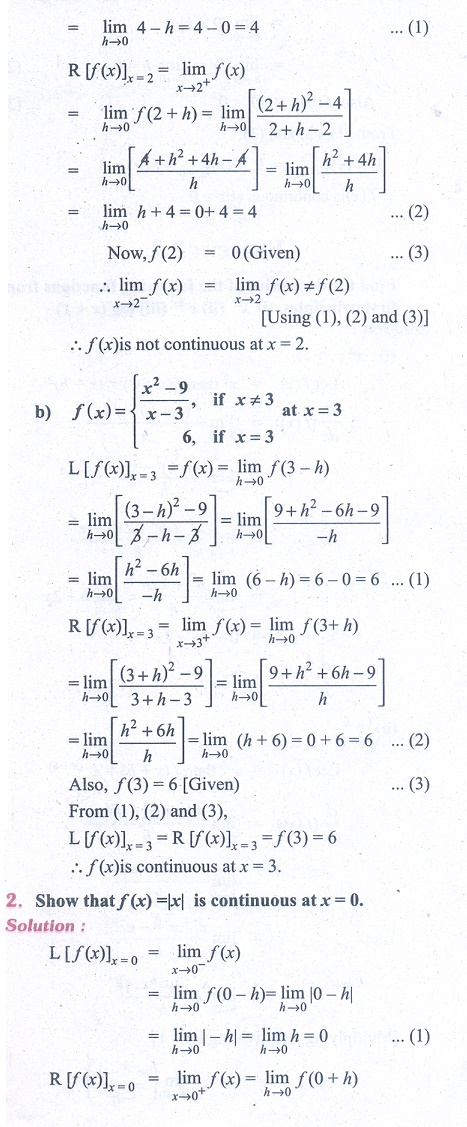Home | | Business Maths 11th std | Exercise 5.3: Limits: continuous functions

# Exercise 5.3: Limits: continuous functions

Business Mathematics and Statistics Book back answers and solution for Exercise questions - Mathematics: Differential Calculus: Limits: continuous functionsTags : Problem Questions with Answer, Solution | Differential Calculus | Mathematics , 11th Business Mathematics and Statistics(EMS) : Chapter 5 : Differential Calculus
Study Material, Lecturing Notes, Assignment, Reference, Wiki description explanation, brief detail
11th Business Mathematics and Statistics(EMS) : Chapter 5 : Differential Calculus : Exercise 5.3: Limits: continuous functions | Problem Questions with Answer, Solution | Differential Calculus | Mathematics